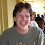## Monday, September 26, 2022

### Is the complexity of approximating Vertex Cover of degree 3 open? (ADDED LATER-NO)

RECALL:

A max-problem f has a A P Time App Scheme (PTAS) if there is an algorithm ALG such that

ALG(\epsilon) \ge (1-\epsilon)f(x).

A min-problem f has a A P Time App Scheme (PTAS) if there is an algorithm ALG such that

ALG(\epsilon) \le (1+\epsilon)f(x).

(Note that the poly can depend on epsilon so it may be something like n^{1/epsilon}.)

MAX3SAT is, given a formula with \le 3 literals per clause, find an assignment

that maximized the number of clauses satisfied.

VCB-a is Vertex cover where graphs have degree \le a

The following are known:

0) MAX3SAT is in APX.

1) The PCP paper, here, showed that if MAX3SAT has a PTAS then P=NP.

2) Papadimitriou and Yannakakis (here)  had showed much earlier that MAX3SAT \le VCB-4 with an approx preserving reduction.

3) From (1) and (2) we have that VCB-4 has a PTAS then P=NP. (VC is in APX by an easy 2-approx).

4) Clearly VCB-2 is in P.

Is VCB-3 a) in P? b) NPC? (ADDED LATER- NPC- See comments.)

Is the following true: if VCB-3 has a PTAS then P=NP. (ADDED LATER- NO PTAS-See Comments)

NOTE- all of the above is true for Ind Set-4 and Dom Set-4. So that leads to more open problems.

1.Vertex Cover on graphs of degree 3 is NP-Complete. This was proven in 1974 by Garey, Johnson and Stockmeyer in the paper "Some simplified NP-complete problems", in their Theorem 2.4.

1.Great, thanks. I think the PTAS problem really is open but would be happy to be proven wrong.

2.If one begins the reduction of the above-mentioned Thm 2.4 from Max 3SAT where each variable occurs a bounded number of times, the same reduction probably establishes APX-hardness with a more careful soundness argument?

3.The problem is APX-hard, as proved in https://doi.org/10.1016/S0304-3975(98)00158-3

A more detailed, but not updated, discussion is at https://www.csc.kth.se/~viggo/wwwcompendium/node10.html

4.THANKS to both Venkat and Anonymous for the references! Google will never replace asking around!

5.This post - and another recent one - have made me think you should consider using MathJax so that the LaTeX you use in your posts can actually render attractively, instead of requiring the reader to parse it